# Installation

The development version can be installed from GitHub with:

# install.packages("devtools")
devtools::install_github("sebastien-plutniak/archeofrag")

Note that Archeofrag requires the RBGL package available through Bioconductor:

if (!requireNamespace("BiocManager", quietly = TRUE))
install.packages("BiocManager")
BiocManager::install("RBGL")

# Building the fragmentation graph

The Archeofrag package comes with a small example data set called “Liang Abu”, related to the pottery fragments found on the surface and in the first two layers of the Liang Abu rock shelter. The data set contains three data frames:

• a table with information about the fragments (a unique identifier, the layer, their length and width, etc.),
• a table with the connection relationships between these fragments (each row contains the unique identifiers of two refitting fragments),
• a table with the similarity relationships between these fragments (two fragments are termed “similar” if they seem to come from the same object but do not have connecting edges.

The make_frag_object function builds objects with the class “frag”. Frag objects are not required by the other Archeofrag functions, however, using them ensures that the data are suitable for the next steps of the analysis. The make_cr_graph function takes a frag object and generates an igraph graph object representing the connection relationships.

library(archeofrag)
data(LiangAbu)
abu.frag <- make_frag_object(cr=df.cr, fragments=fragments.info)
abu.g <- make_cr_graph(abu.frag)

# Visualisation and subgraph extraction

Several Archeofrag functions ensure that the first examination of the data is easy. The frag.relations.by.layers function returns a matrix with the number of relationships within and between spatial units (e.g., stratigraphic layer).

frag.relations.by.layers(abu.g, "layer")
#>
#>      0  1  2
#>   0  4
#>   1  0 18
#>   2  0  3 31

The diagonal of the matrix gives the number of intra-layer relationships, and the other values refer to inter-layer relationships. Here, for example, there are 31 connection relationships within layer 2, and 3 connection relationships between layers 1 and 2. No connection relationship was found between the surface (“0”) and layer 2.

The frag.graph.plot function generates a visual representation of the graph:

par(mar=c(1, 0, 2, 0))
frag.graph.plot(abu.g, layer.attr="layer", main="All layers")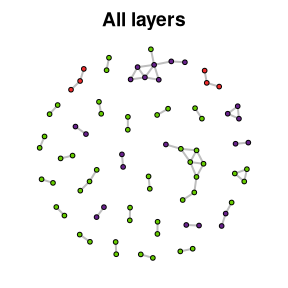The fragments are coloured by layer and the three inter-layer relationships can be observed.

Let us now focus on layers 1 and 2. The frag.get.layers.pair function allows the user to extract a pair of layers.

abu.g12 <- frag.get.layers.pair(abu.g, layer.attr="layer",
sel.layers=c("1", "2"))

This subgraph is drawn with the frag.graph.plot function:

par(mar=c(1, 0, 2, 0))
frag.graph.plot(abu.g12, layer.attr="layer", main="Layers 1 and 2")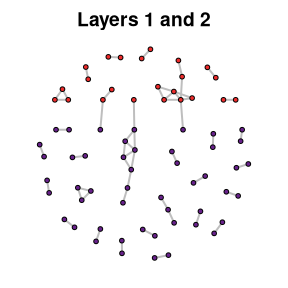The function has a different behaviour if applied to a fragmentation graph with only two spatial units: the nodes are vertically localised to reflect their location in the two spatial units. In addition, note that standard plot arguments can be passed to the frag.graph.plot function, e.g., the main argument to define the plot’s title.

The frag.get.layers.pair function has additional parameters to set the minimum size of the connected fragments sets (size.mini) and to extract only the sets of connected fragments which include relationships between the two spatial units (mixed.components.only).

frag.get.layers.pair(abu.g, layer.attr="layer", sel.layers=c("1", "2"),
size.mini=2, mixed.components.only=TRUE)
#> IGRAPH fded981 UN-- 19 22 --
#> + attr: frag_type (g/c), name (v/c), layer (v/c), zmin (v/n), zmax
#> | (v/n), square (v/c), sherd.type (v/c), thickness (v/n), length (v/n),
#> | membership (v/n), type_relation (e/c)
#> + edges from fded981 (vertex names):
#>   187--188 165--195 195--196 195--197 196--198 195--204 196--204 197--204
#>   198--204 195--25  188--250 27 --28  27 --366 27 --367 28 --367 366--367
#>  27 --371 332--371 366--371 25 --8   28 --835 835--836

Additionally, the frag.get.layers function can extract a set of specified spatial unit(s), e.g., the refits within the first layer at Liang Abu:

frag.get.layers(abu.g, layer.attr="layer", sel.layers="1")
#> $1 #> IGRAPH 4177d33 UN-- 23 18 -- #> + attr: frag_type (g/c), name (v/c), layer (v/c), zmin (v/n), zmax #> | (v/n), square (v/c), sherd.type (v/c), thickness (v/n), length (v/n), #> | type_relation (e/c) #> + edges from 4177d33 (vertex names): #>  123--124 187--188 195--196 195--197 196--198 195--204 196--204 #>  197--204 198--204 195--25 301--302 313--314 392--408 435--441 #>  477--478 25 --8 435--9999 441--9999 # Edge weighting, cohesion and admixture computation Weighting the edges is a crucial step in the TSAR / Archeofrag approach because it integrates the topological properties of the fragmentation graph. The frag.edges.weighting function assigns a value to each edge based on the topological properties of the vertices this edge connects. abu.g12 <- frag.edges.weighting(abu.g12, layer.attr="layer") Then, the frag.layers.cohesion function is used to calculate the cohesion value of each layer. frag.layers.cohesion(abu.g12, layer.attr="layer") #> cohesion1 cohesion2 #> 1/2 0.3977727 0.5927749 These values determine the cohesion (self-adherence) of the spatial units (here, layers) based on the distribution of the refitting relationships. Note that the weighting of the edges is mandatory for the computation of cohesion. Using the frag.layers.cohesion function on a non-weighted fragmentation graph will give an error. In addition to topological properties, the computation of edge weights can optionally include other parameters, namely the morphometry of the fragments and the distance between the location where they were found. In the following example, the length of the pottery sherds is used as a morphometric proxy: abu.g12morpho <- frag.edges.weighting(abu.g12, layer.attr="layer", morphometry="length") Using the morphometry parameter results, layer 2 is more cohesive than layer 1: frag.layers.cohesion(abu.g12morpho, layer.attr="layer") #> cohesion1 cohesion2 #> 1/2 0.3263172 0.666898 In addition, the frag.layers.admixture function returns a value quantifying the admixture between the two layers. Let us compare the results obtained when the morphometry is used or not: # topology-based weighting: frag.layers.admixture(abu.g12, layer.attr="layer") #> admixture #> 0.009452435 # topology + morphometry weighting: frag.layers.admixture(abu.g12morpho, layer.attr="layer") #> admixture #> 0.006784769 In this case, using the morphometry in the computation lowers the admixture between layers 1 and 2 at Liang Abu. # Testing layer formation hypotheses using simulated data Simulation-based hypotheses can be tested by combining the functions offered by Archeofrag. ## Generating artificial fragmentation graphs The frag.simul.process function generates a pair of spatial units containing fragmented objects with connection relationships within and between these units. The next command creates two spatial units populated with 20 initial objects (corresponding to the “connected components” of a graph) which are fragmented into 50 pieces. simul.g <- frag.simul.process(n.components=20, vertices=50) This illustrates the simplest use of the frag.simul.process function, which has several other parameters to control the features of the simulation. The number of initial spatial units is a crucial parameter, set using the initial.layers parameter with “1” or “2”. This parameter determines the method used to construct the graph and, accordingly, the underlying formation process hypothesis. If initial.layers is “1”, the fragmentation process is simulated assuming that all the objects were originally buried in a single spatial unit. The two clusters observed at the end of the process are due to fragmentation and displacement. 1. A single spatial unit is populated with the initial objects, 2. the fragmentation process is applied, 3. spatial units are assigned to the fragments, 4. some fragments are moved as determined by the value of the disturbance parameter. If initial.layers is “2”, it assumes that the objects were buried in two different spatial units, which were later partially mixed due to fragmentation and displacement: 1. two spatial units are populated with the initial objects (components), 2. the fragmentation process is applied, 3. disturbance is applied. The vertices and edges parameters are related: at least one of them must be set, or both (only if initial.layers is set to 1). Note that using both parameters at the same time increases the constraints and reduces the number of possible solutions to generate the graph. When there is no solution, an error occurs and a message suggests how to change the parameters. The balance argument determines the number of fragments in the smaller spatial unit (before the application of the disturbance process). The components.balance also determines the contents of the two spatial units by affecting the distribution of the initial objects (components). Note that this argument is used only when initial.layers is set to 2. The aggreg.factor parameter affects the distribution of the sizes of the components: this distribution tends to be more unequal when aggreg.factor has values close to 1. By default, fragments from two spatial units can be disturbed and moved to another other spatial unit. However, the asymmetric.transport.from can be used to move fragments from only one given spatial unit. Finally, the planar argument determines if the generated graph has to be planar or not (a graph is planar when it can be drawn on a plane, without edges crossing). This function requires to install the optional RBGL package. An example of a complete configuration of the function is: frag.simul.process(initial.layers=1, n.components=20, vertices=50, edges=40, balance=.4, components.balance=.4, disturbance=.1, aggreg.factor=0, planar=FALSE, asymmetric.transport.from="1") An additional function is intended to simulate the failure of an observer to determine the relationships between fragments. The frag.observer.failure function takes a fragmentation graph and randomly removes a given proportion of edges. frag.observer.failure(abu.g12, likelihood=0.2) ## Testing hypotheses The versatile frag.simul.process function can generate fragmentation graphs under multiple hypotheses about the initial conditions (number of initial objects, number of initial spatial units, etc.). Testing measurements on observed empirical data against measurements made under these hypotheses can determine the most likely initial conditions and fragmentation process. Here, this is illustrated by comparing measurements from Liang Abu layers 1 and 2 with measurements from simulated data under two hypotheses about the number of initial spatial units (e.g., layers), using the initial.layers parameter with two values, namely one or two initial layers. A fragmentation graph is generated for each initial.layers value, using the parameters observed in the Liang Abu layers 1 and 2 fragmentation graph. Setting the simulator is made easier by using the frag.get.parameters function, which takes a graph and computes a series of parameters that are returned as a list. params <- frag.get.parameters(abu.g12, layer.attr="layer") params #>$n.components
#>  28
#>
#> $vertices #>  72 #> #>$edges
#>  52
#>
#> $balance #>  0.32 #> #>$components.balance
#>  0.29
#>
#> $disturbance #>  0.04 #> #>$aggreg.factor
#>  0.7
#>
#> $planar #>  TRUE # for H2: test.2layers.g <- frag.simul.process(initial.layers=2, n.components=params$n.components,
vertices=params$vertices, disturbance=params$disturbance,
aggreg.factor=params$aggreg.factor, planar=params$planar)
# for H1:
test.1layer.g <- frag.simul.process(initial.layers=1,
n.components=params$n.components, vertices=params$vertices,
disturbance=params$disturbance, aggreg.factor=params$aggreg.factor,
planar=params$planar) Let us now generate not only one graph, but a large number of graphs to statistically compare measurements in the empirical and simulated graphs. The frag.simul.process function is set for the “two initial layers” hypothesis and embedded into an ad hoc function: run.test2 <- function(x){ frag.simul.process(initial.layers=2, # note the different value n.components=params$n.components,
vertices=params$vertices, disturbance=params$disturbance,
aggreg.factor=params$aggreg.factor, planar=params$planar)
}

The function is then executed a sufficient number of times:

test2.results <- lapply(1:100, run.test2)

The empirical values observed for Liang Abu layers 1 and 2 (red line) can now be compared to the values measured in the simulated graph generated under the hypothesis of two initial layers. This shows, for example, that the empirical admixture value is slightly lower than the simulated admixture values:

edges.res <- sapply(test2.results,
function(g) frag.get.parameters(g, "layer")$edges) plot(stats::density(edges.res), main="Edges") abline(v=params$edges, col="red")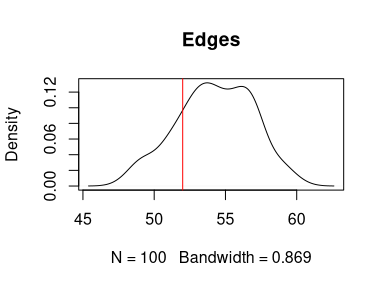Similarly, the empirical admixture value is lower than the simulated admixture values:

admix.res <- sapply(test2.results,
abline(v=frag.layers.admixture(abu.g12, "layer"), col="red")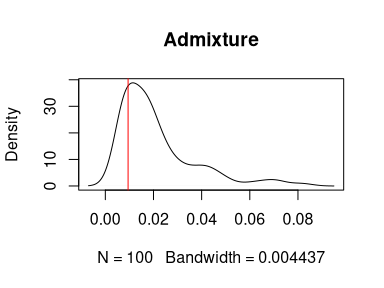Two functions (frag.simul.compare and frag.simul.summarise) facilitate the execution of the analytical process described above on the initial number of spatial units. The frag.simul.compare function takes an observed fragmentation graph, generates two series of simulated graphs corresponding to two hypotheses on the number of initial spatial units (H1 for one initial spatial unit and H2 for two initial spatial units), and returns a data frame of measurements made on each series (including the edge count, weights sum, balance value, disturbance value, admixture value, and cohesion values of the two spatial units).

compare.res <- frag.simul.compare(abu.g12, layer.attr="layer",
iter=30, summarise=FALSE)
head(compare.res$h1.data) #> edges weightsum balance disturbance admixture cohesion1 cohesion2 #> 1 56 276.2984 0.2916667 0.05357143 0.007656271 0.4032302 0.5891135 #> 2 54 201.2558 0.3333333 0.11111111 0.040275001 0.4317919 0.5279331 #> 3 54 221.4090 0.3472222 0.09259259 0.024917846 0.3592728 0.6158094 #> 4 52 205.4636 0.3611111 0.09615385 0.026349836 0.3443625 0.6292877 #> 5 48 151.2775 0.3194444 0.06250000 0.011943286 0.2252171 0.7628396 #> 6 55 252.2477 0.3333333 0.07272727 0.015046719 0.4254303 0.5595230 For each of these parameters, the frag.simul.summarise function facilitates the comparison between empirical observed values and simulated values generated for H1 and H2. frag.simul.summarise(abu.g12, layer.attr="layer", compare.res$h1.data,
compare.res$h2.data) #> H1 != H2? p.value Obs. value/H1 Obs. value/H2 #> edges FALSE 0.15 lower lower #> weightsum FALSE 0.5 lower lower #> balance FALSE 0.16 within lower #> disturbance FALSE 0.64 lower lower #> admixture FALSE 0.95 within within #> cohesion1 TRUE 0 higher within #> cohesion2 TRUE 0 lower within This function returns a data frame with four columns, containing, for each parameter studied: 1. whether the series of H1 values are statistically different to the H2 series (Boolean), 2. the p-value of the Wilcoxon test (numerical), 3. whether the observed value is “within”, “higher”, or “lower” to the interquartile range of values for H1, 4. whether the observed value is “within”, “higher”, or “lower” to the interquartile range of values for H2. Note that the frag.simul.compare function can optionally be set to execute and return the results of the frag.simul.summarise function. # Assessing spatial unit boundaries using similarity relationships Similarity relationships are, by construction, not part of the TSAR method, which is based on the topological properties of connection networks. However, since similarity relationships are more frequent in archaeological empirical studies, the Archeofrag package includes various functions to handle them. This section illustrates a method to use similarity relationships using Archeofrag and R generic functions. The make_sr_graph function takes a “frag” object and generates an igraph similarity network. # make a frag object and generate a similarity graph: abu.frag <- make_frag_object(sr=df.sr, fragments=fragments.info) abu.sr <- make_sr_graph(abu.frag) The frag.relations.by.layers function returns a table with the number of similarity relationships in and between spatial units, e.g., in the top three layers at Liang Abu: # count of similarity relationships in and between layers: simil.by.layers.df <- frag.relations.by.layers(abu.sr, "layer") simil.by.layers.df #> #> 0 1 2 #> 0 15 #> 1 0 234 #> 2 1 61 173 These values can be observed as percentages: # percentage of similarity relationships in and between layers: round(simil.by.layers.df / sum(simil.by.layers.df, na.rm=T) * 100, 0) #> #> 0 1 2 #> 0 3 #> 1 0 48 #> 2 0 13 36 Considering a stratigraphic sequence, adjacent and close layers in the sequence must have lower statistical distances than distant layers. Consequently, it is expected that the result of a hierarchical clustering computed on this distance table would reflect the order of the layers. The expected result is observed for Liang Abu surface and the first two layers, suggesting an absence of significant disturbance and admixture (). # turn similarity into distance: simil.dist <- max(c(simil.by.layers.df), na.rm=T) - simil.by.layers.df simil.dist <- as.dist(simil.dist) # hierarchical clustering: clust.res <- stats::hclust(simil.dist, method="ward.D2") clust.res$labels <- as.character(factor(clust.res\$labels,
levels=c("0", "1", "2"),
labels=c("layer 0", "layer 1", "layer 2")))
plot(clust.res, hang=-1, axes=F, ann=F)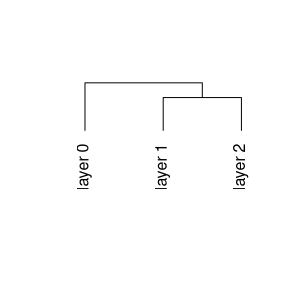Hierarchical clustering of the pottery layers in Liang Abu (distance: based on the number of similarity relationships; clustering method: Ward).

# Characterising spatial units from their fragmentation

The second aim of the TSAR method implemented in Archeofrag is to characterise spatial units based on the topological properties of the connection relationships between the fragments they contain. Although this aspect is still a work in progress, some functions are already implemented and will be illustrated using simulated data. The archaeological interpretation of numerical values depends on the type of material (lithic, pottery, etc.) and the completeness or incompleteness of the objects under study and is not discussed here.

# simulate a fragmentation graph:
simul.g <- frag.simul.process(initial.layers=2,
n.components=20,
vertices=70,
balance=.45)
# extract the subgraph of each spatial unit:
simul.g1 <- frag.get.layers(simul.g, layer.attr="layer", sel.layers="1")[]
simul.g2 <- frag.get.layers(simul.g, layer.attr="layer", sel.layers="2")[]

In a graph, a cycle is a path in which only the first and last vertices are repeated. The frag.cycles function searches for cycles in a graph and returns the number of cycles found for different cycle lengths. The kmax parameter determines the maximal length of the cycles to search for. Let us compare the cycles found in the two spatial units of the artificial graph:

rbind(
"unit1" = frag.cycles(simul.g1, kmax=5),
"unit2" = frag.cycles(simul.g2, kmax=5))
#>       3-cycles 4-cycles 5-cycles
#> unit1       10        1        0
#> unit2       20       11        3

The frag.path.lengths function returns the distribution of the path lengths in the graph (i.e., the number of edges between each pair of vertices). This function returns a vector whose first element is the frequency of the paths of length 1, the second element is the frequency of the paths of length 2, etc. If the cumulative parameter is set to TRUE, the function returns the cumulative relative frequency of the path lengths.

frag.path.lengths(simul.g1)
#>  31  7
frag.path.lengths(simul.g2)
#>  45 14  1
frag.path.lengths(simul.g2, cumulative=T)
#>  1.00000000 0.31111111 0.02222222

In a graph, the shortest path between two vertices is the path including the least number of edges. The diameter of a graph is its longest shortest path. The frag.diameters function calculates the diameter of each component of the graph and returns the frequency of the values. If the cumulative parameter is set to TRUE, the function returns the cumulative relative frequency of the diameters.

frag.diameters(simul.g1)
#> 1 2
#> 6 4
frag.diameters(simul.g2)
#> 1 2 3
#> 3 6 1